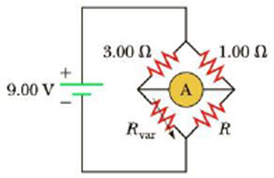Chapter 18, Problem 19P

Chapter
Section
Textbook Problem

Figure P18.19 shows a Wheatstone bridge, a circuit used to precisely measure an unknown resistance R by varying Rvar until the ammeter reads zero current and the bridge is said to be “balanced.” If the bridge is balanced with Rvar = 9.00 Ω, find (a) the value of the unknown resistance Rand (b) the current in the 1.00 Ω resistor. (Hint: With the bridge balanced, the wire through the ammeter can effectively be removed from the circuit, leaving two pairs of resistors in parallel.)Figure Pl8.19

(a)

To determine
The value of the unknown resistance R.

Explanation

Given Info: The variable resistance is Rvar=9.00Ω .

Explanation:

The given figure shows the balanced Wheatstone bridge network, if the given circuit balanced by varying the resistance until the ammeter shows zero current, and the wire through the ammeter is an equipotential.

Consider I1 as the current flows in the left branch of the Wheatstone circuit and I2 as the current flows in the right branch both current shows in the downward direction.

From the Kirchhoff’s loop rule, the current moving clockwise around the top and bottom will separate the bridge into two halves.

From the Kirchhoff’s loop rule at the top of the bridge.

(1.00Ω)I2+(3.00Ω)I1=0 (1)

• I1 and I2 is the current flows through the left and right branch of the bridge network,

From the Kirchhoff’s loop rule at the bottom of the bridge.

RI2+RvarI1=0 (2)

• R is the unknown resistance,
• Rvar is the variable resistance,

Substitute 9.00Ω for Rvar in the equation (2).

RI2+(9

(b)

To determine
The current in the 1.00Ω resistor.

Still sussing out bartleby?

Check out a sample textbook solution.

See a sample solution

The Solution to Your Study Problems

Bartleby provides explanations to thousands of textbook problems written by our experts, many with advanced degrees!

Get Started

Find more solutions based on key concepts